# PostgreSQL Tutorial: Window Functions

September 26, 2023

Summary: in this tutorial, you will learn how to use the PostgreSQL window functions to perform the calculation across a set of rows related to the current row.

## Setting up sample tables

First, create two tables named `products` and `product_groups` for the demonstration: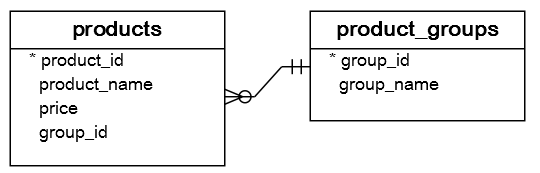``````CREATE TABLE product_groups (
group_id serial PRIMARY KEY,
group_name VARCHAR (255) NOT NULL
);

CREATE TABLE products (
product_id serial PRIMARY KEY,
product_name VARCHAR (255) NOT NULL,
price DECIMAL (11, 2),
group_id INT NOT NULL,
FOREIGN KEY (group_id) REFERENCES product_groups (group_id)
);
``````

Second, insert some rows into these tables:

``````INSERT INTO product_groups (group_name)
VALUES
('Smartphone'),
('Laptop'),
('Tablet');

INSERT INTO products (product_name, group_id,price)
VALUES
('Microsoft Lumia', 1, 200),
('HTC One', 1, 400),
('Nexus', 1, 500),
('iPhone', 1, 900),
('HP Elite', 2, 1200),
('Sony VAIO', 2, 700),
('Dell Vostro', 2, 800),
('Kindle Fire', 3, 150),
('Samsung Galaxy Tab', 3, 200);
``````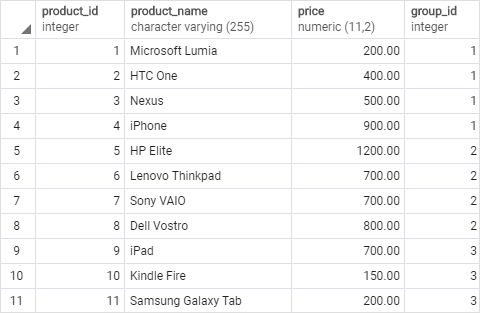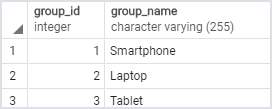## Introduction to PostgreSQL window functions

The easiest way to understand the window functions is to start by reviewing the aggregate functions. An aggregate function aggregates data from a set of rows into a single row.

The following example uses the `AVG()` aggregate function to calculate the average price of all products in the `products` table.

``````SELECT
AVG (price)
FROM
products;
``````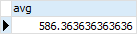To apply the aggregate function to subsets of rows, you use the `GROUP BY` clause. The following example returns the average price for every product group.

``````SELECT
group_name,
AVG (price)
FROM
products
INNER JOIN product_groups USING (group_id)
GROUP BY
group_name;
``````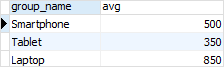As you see clearly from the output, the `AVG()` function reduces the number of rows returned by the queries in both examples.

Similar to an aggregate function, a window function operates on a set of rows. However, it does not reduce the number of rows returned by the query.

The term window describes the set of rows on which the window function operates. A window function returns values from the rows in a window.

For instance, the following query returns the product name, the price, product group name, along with the average prices of each product group.

``````SELECT
product_name,
price,
group_name,
AVG (price) OVER (
PARTITION BY group_name
)
FROM
products
INNER JOIN
product_groups USING (group_id);
``````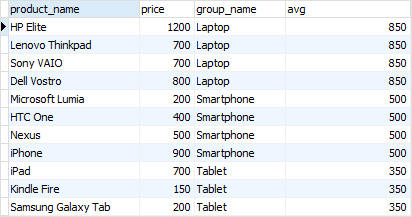In this query, the `AVG()` function works as a window function that operates on a set of rows specified by the `OVER` clause. Each set of rows is called a window.

The new syntax for this query is the `OVER` clause:

``````AVG(price) OVER (PARTITION BY group_name)
``````

In this syntax, the `PARTITION BY` distributes the rows of the result set into groups and the `AVG()` function is applied to each group to return the average price for each.

Note that a window function always performs the calculation on the result set after the `JOIN`, `WHERE`, `GROUP BY` and `HAVING` clause and before the final `ORDER BY` clause in the evaluation order.

## PostgreSQL Window Function Syntax

PostgreSQL has a sophisticated syntax for window function call. The following illustrates the simplified version:

``````window_function(arg1, arg2,..) OVER (
[PARTITION BY partition_expression]
[ORDER BY sort_expression [ASC | DESC] [NULLS {FIRST | LAST }])
``````

In this syntax:

### `window_function(arg1,arg2,...)`

The `window_function` is the name of the window function. Some window functions do not accept any argument.

### `PARTITION BY` clause

The `PARTITION BY` clause divides rows into multiple groups or partitions to which the window function is applied. Like the example above, we used the product group to divide the products into groups (or partitions).

The `PARTITION BY` clause is optional. If you skip the `PARTITION BY` clause, the window function will treat the whole result set as a single partition.

### `ORDER BY` clause

The `ORDER BY` clause specifies the order of rows in each partition to which the window function is applied.

The `ORDER BY` clause uses the `NULLS FIRST` or `NULLS LAST` option to specify whether nullable values should be first or last in the result set. The default is `NULLS LAST` option.

### `frame_clause`

The `frame_clause` defines a subset of rows in the current partition to which the window function is applied. This subset of rows is called a frame.

If you use multiple window functions in a query:

``````SELECT
wf1() OVER(PARTITION BY c1 ORDER BY c2),
wf2() OVER(PARTITION BY c1 ORDER BY c2)
FROM table_name;
``````

you can use the `WINDOW` clause to shorten the query as shown in the following query:

``````SELECT
wf1() OVER w,
wf2() OVER w,
FROM table_name
WINDOW w AS (PARTITION BY c1 ORDER BY c2);
``````

It is also possible to use the `WINDOW` clause even though you call one window function in a query:

``````SELECT wf1() OVER w
FROM table_name
WINDOW w AS (PARTITION BY c1 ORDER BY c2);
``````

## PostgreSQL window function List

The following table lists all window functions provided by PostgreSQL. Note that some aggregate functions such as `AVG()`, `MIN()`, `MAX()`, `SUM()`, and `COUNT()` can be also used as window functions.

Name Description
CUME_DIST Return the relative rank of the current row.
DENSE_RANK Rank the current row within its partition without gaps.
FIRST_VALUE Return a value evaluated against the first row within its partition.
LAG Return a value evaluated at the row that is at a specified physical offset row before the current row within the partition.
LAST_VALUE Return a value evaluated against the last row within its partition.
LEAD Return a value evaluated at the row that is `offset `rows after the current row within the partition.
NTILE Divide rows in a partition as equally as possible and assign each row an integer starting from 1 to the argument value.
NTH_VALUE Return a value evaluated against the nth row in an ordered partition.
PERCENT_RANK Return the relative rank of the current row (rank-1) / (total rows – 1)
RANK Rank the current row within its partition with gaps.
ROW_NUMBER Number the current row within its partition starting from 1.

## The `ROW_NUMBER()`, `RANK()`, and `DENSE_RANK()` functions

The `ROW_NUMBER()`, `RANK()`, and `DENSE_RANK()` functions assign an integer to each row based on its order in its result set.

The `ROW_NUMBER()` function assigns a sequential number to each row in each partition. See the following query:

``````SELECT
product_name,
group_name,
price,
ROW_NUMBER () OVER (
PARTITION BY group_name
ORDER BY
price
)
FROM
products
INNER JOIN product_groups USING (group_id);
``````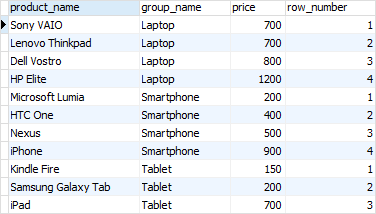The `RANK()` function assigns ranking within an ordered partition. If rows have the same values, the `RANK()` function assigns the same rank, with the next ranking(s) skipped.

See the following query:

``````SELECT
product_name,
group_name,
price,
RANK () OVER (
PARTITION BY group_name
ORDER BY
price
)
FROM
products
INNER JOIN product_groups USING (group_id);
``````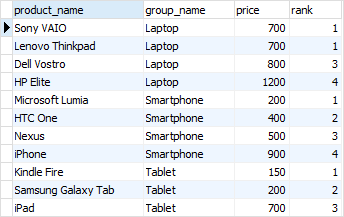In the laptop product group, both `Dell Vostro` and `Sony VAIO` products have the same price, therefore, they receive the same rank 1. The next row in the group is `HP Elite` that receives the rank 3 because the rank 2 is skipped.

Similar to the `RANK()` function, the `DENSE_RANK()` function assigns a rank to each row within an ordered partition, but the ranks have no gap. In other words, the same ranks are assigned to multiple rows and no ranks are skipped.

``````SELECT
product_name,
group_name,
price,
DENSE_RANK () OVER (
PARTITION BY group_name
ORDER BY
price
)
FROM
products
INNER JOIN product_groups USING (group_id);
``````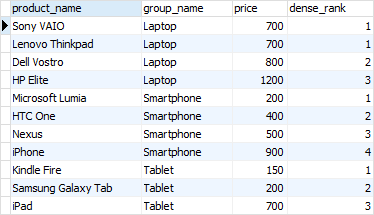Within the laptop product group, rank 1 is assigned twice to `Dell Vostro` and `Sony VAIO`. The next rank is 2 assigned to `HP Elite`.

## The `FIRST_VALUE` and `LAST_VALUE` functions

The `FIRST_VALUE()` function returns a value evaluated against the first row within its partition, whereas the `LAST_VALUE()` function returns a value evaluated against the last row in its partition.

The following statement uses the `FIRST_VALUE()` to return the lowest price for every product group.

``````SELECT
product_name,
group_name,
price,
FIRST_VALUE (price) OVER (
PARTITION BY group_name
ORDER BY
price
) AS lowest_price_per_group
FROM
products
INNER JOIN product_groups USING (group_id);
``````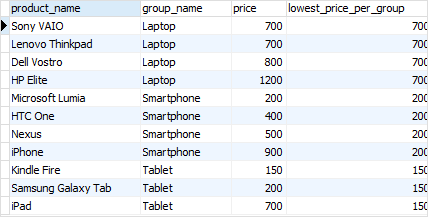The following statement uses the `LAST_VALUE()` function to return the highest price for every product group.

``````SELECT
product_name,
group_name,
price,
LAST_VALUE (price) OVER (
PARTITION BY group_name
ORDER BY
price RANGE BETWEEN UNBOUNDED PRECEDING
AND UNBOUNDED FOLLOWING
) AS highest_price_per_group
FROM
products
INNER JOIN product_groups USING (group_id);
``````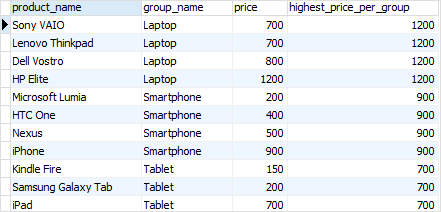Notice that we added the frame clause `RANGE BETWEEN UNBOUNDED PRECEDING AND UNBOUNDED FOLLOWING` because by default the frame clause is `RANGE BETWEEN UNBOUNDED PRECEDING AND CURRENT ROW`.

## The `LAG` and `LEAD` functions

The `LAG()` function has the ability to access data from the previous row, while the `LEAD()` function can access data from the next row.

Both `LAG()` and `LEAD()` functions have the same syntax as follows:

``````LAG  (expression [,offset] [,default]) over_clause;
``````

In this syntax:

• `expression` – a column or expression to compute the returned value.
• `offset` – the number of rows preceding ( `LAG`)/ following ( `LEAD`) the current row. It defaults to 1.
• `default` – the default returned value if the `offset` goes beyond the scope of the window. The `default` is `NULL` if you skip it.

The following statement uses the `LAG()` function to return the prices from the previous row and calculates the difference between the price of the current row and the previous row.

``````SELECT
product_name,
group_name,
price,
LAG (price, 1) OVER (
PARTITION BY group_name
ORDER BY
price
) AS prev_price,
price - LAG (price, 1) OVER (
PARTITION BY group_name
ORDER BY
price
) AS cur_prev_diff
FROM
products
INNER JOIN product_groups USING (group_id);
``````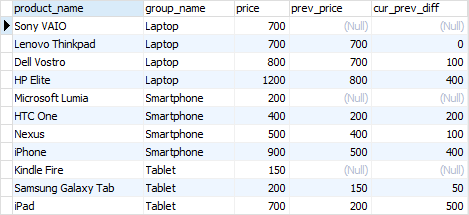The following statement uses the `LEAD()` function to return the prices from the next row and calculates the difference between the price of the current row and the next row.

``````SELECT
product_name,
group_name,
price,
PARTITION BY group_name
ORDER BY
price
) AS next_price,
price - LEAD (price, 1) OVER (
PARTITION BY group_name
ORDER BY
price
) AS cur_next_diff
FROM
products
INNER JOIN product_groups USING (group_id);
``````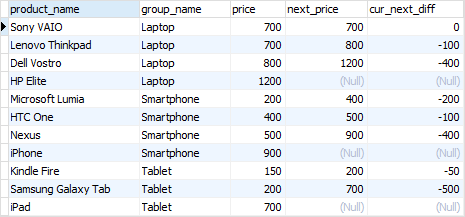In this tutorial, we have introduced you to the PostgreSQL window functions and shown you some examples of using them to query data.